Question

a. How many moles of xenon trioxide are present in 4.95 x 1022 molecules of this...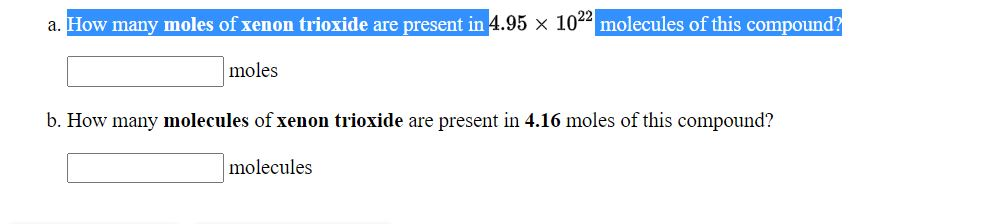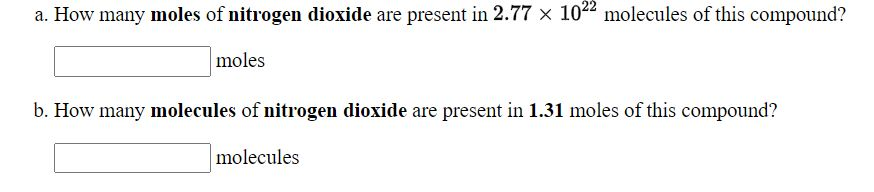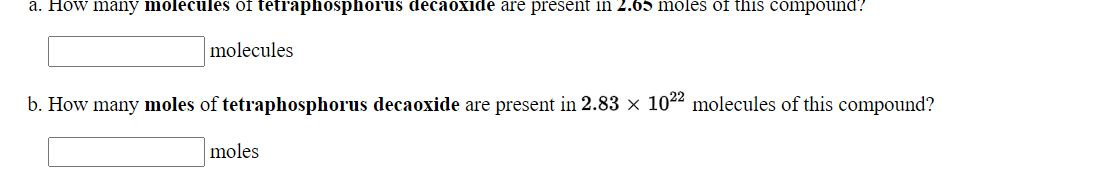a. How many moles of xenon trioxide are present in 4.95 x 1022 molecules of this compound? moles b. How many molecules of xenon trioxide are present in 4.16 moles of this compound? molecules
a. How many moles of nitrogen dioxide are present in 2.77 x 1022 molecules of this compound? moles b. How many molecules of nitrogen dioxide are present in 1.31 moles of this compound? molecules
a. How many molecules of tetraphosphorus decaoxide are present in 2.05 moles of this compound? molecules b. How many moles of tetraphosphorus decaoxide are present in 2.83 x 1022 molecules of this compound? moles

We need at least 10 more requests to produce the answer.

0 / 10 have requested this problem solution

The more requests, the faster the answer.

All students who have requested the answer will be notified once they are available.

Earn Coins

Coins can be redeemed for fabulous gifts.

Similar Homework Help Questions
• a. How many molecules of tetraphosphorus decaoxide are present in 2.96 moles of this compound? 1783x10^23...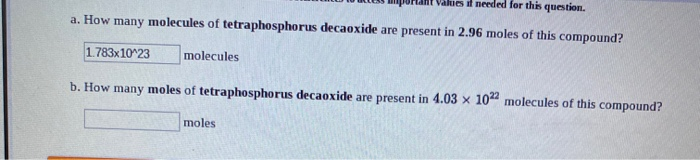a. How many molecules of tetraphosphorus decaoxide are present in 2.96 moles of this compound? 1783x10^23 molecules b. How many moles of tetraphosphorus decaoxide are present in 4.03 x 10" molecules of this compound? moles

• how many molecules of tetraphosphorus decaoxide are present in 4.31 moles of this compound ? and...

how many molecules of tetraphosphorus decaoxide are present in 4.31 moles of this compound ? and how many moles of tetraphosphorus decaoxide are present in 1.07x10^22 molecules of this compound ?

• The formula for xenon trioxide is . a. How many molecules are in 6.56 grams of...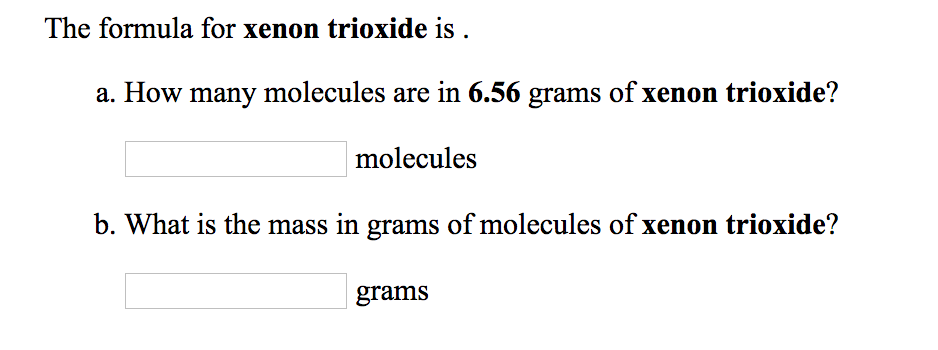The formula for xenon trioxide is . a. How many molecules are in 6.56 grams of xenon trioxide? molecules b. What is the mass in grams of molecules of xenon trioxide? grams

• 1. How many MOLECULES of carbon tetrabromide are present in 2.48 moles of this compound? molecules....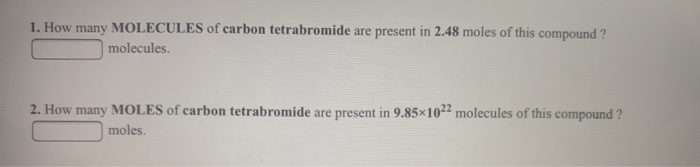1. How many MOLECULES of carbon tetrabromide are present in 2.48 moles of this compound? molecules. 2. How many MOLES of carbon tetrabromide are present in 9.85*1022 molecules of this compound? moles.

• Use the References to access important values if needed for this question. How many ATOMS of...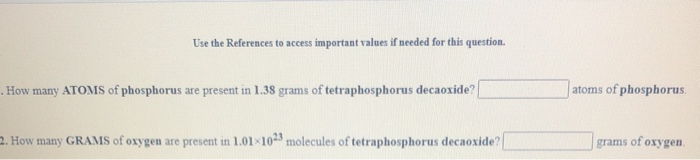Use the References to access important values if needed for this question. How many ATOMS of phosphorus are present in 1.38 grams of tetraphosphorus decaoxide? atoms of phosphorus. 2. How many GRAMS of oxygen are present in 1.01 1023 molecules of tetraphosphorus decaoxide? grams of oxygen days grams 1. How many GRAMS of potassium sulfate are present in 4.96 moles of this compound? moles 2. How many MOLES of potassium sulfate are present in 1.67 grams of this compound?

• 1. How many grams of O are there in a sample of O that contains the...

1. How many grams of O are there in a sample of O that contains the same number of moles as a 55.4 gram sample of Kr? 2. How many MOLECULES of nitrogen dioxide are present in 3.28 grams of this compound 3. . How many GRAMS of nitrogen dioxide are present in 7.63×1022 molecules of this compound

• Use the References to access important values if needed for this question moles 1. How many...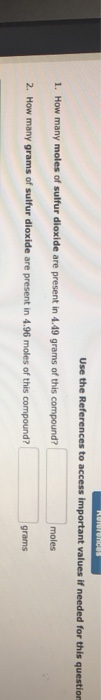Use the References to access important values if needed for this question moles 1. How many moles of sulfur dioxide are present in 4.49 grams of this compound? 2. How many grams of sulfur dioxide are present in 4.96 moles of this compound grams Use the References to access important values if needed for this question How many moles of sulfur dioxide are present in 9.61 × 1022 molecules of this compound? moles kilograms grams milligrams 17.9 56.5

• a. How many moles of sulfur trioxide, SO3, are present in a sample that contains 2.49...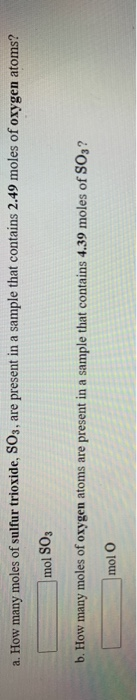a. How many moles of sulfur trioxide, SO3, are present in a sample that contains 2.49 moles of oxygen atoms? mol SO, b. How many moles of oxygen atoms are present in a sample that contains 4.39 moles of SO3? mol

• 45. Refer to the following equation: 4NH3(g) + 70,() → 4NO2()+6H20() How many moles of ammonia...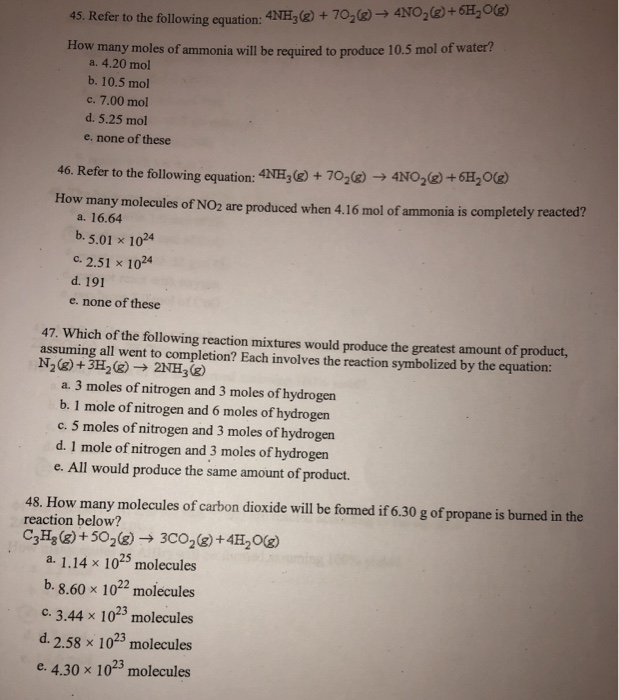45. Refer to the following equation: 4NH3(g) + 70,() → 4NO2()+6H20() How many moles of ammonia will be required to produce 10.5 mol of water a. 4.20 mol b. 10.5 mol c. 7.00 mol d. 5.25 mol e. none of these 46. Refer to the following equation: 4NH3(Ⓡ) + 702() → 4NO2(%) +6H20(g) How many molecules of NO2 are produced when 4.16 mol of ammonia is completely reacted a. 16.64 b. 5.01 X 1024 c. 2.51 1024 d. 191 e....

• How many moles of sodium chloride is equivalent to 6.33 x 1022 NaCl "molecules" (formula units)??...

How many moles of sodium chloride is equivalent to 6.33 x 1022 NaCl "molecules" (formula units)?? Answer using 3 decimal places.